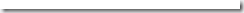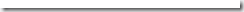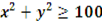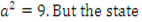### MA2265 Discrete Mathematics Two Mark Questions With Answers 2014

Anna University, ChennaiSub. Code & Subject : MA2265- Discrete Mathematics (DM)

1.Define negation?

Unit-I&II

 Adhiparasakthi College of Engg., G.B. Nagar, Kalavai CD / Page 1 of 10
 MA2265 – Discrete Mathematics GINEERING DEPARTMENT OF COMPUTER SCIENCE & EN
 GINEERING
If p is a statement, then negation of p written as ~p (or ~p } or 7p and read as “not p”. the truth
 p ~p T F F T
table is as follows

Example: P: madras is a city

~p:madras is not a city or it is not the case that madras is a city.

2.define conjunction?

 P Q P^Q T T F F T F T F T F F F
The conjunction if 2 statements P and Q is the statement P^Q which is read as “P and Q”. the statement P^Q has a truth value T whenever both P and Q have the truth table T; otherwise it has truth value F. the conjunction is defined by the truth table as follows.

Example : P : jack went up the hill

Q: jill went up the hill

P^Q: jack and jill went up the hill

3.define disjunction?

The disjunction of 2 statements P and Q is the statement P v Q which is read as “P or Q”. the statement P v Q has a truth value F only when both P and Q have truth value F otherwise it is true. The disjunction is defined by following table.

P Q P v Q

AkAsHViShAl

Example :

T T T T F T F T T F F F

 Adhiparasakthi College of Engg., G.B. Nagar, Kalavai CD / Page 2 of 10
 III Year – V Semester CSE MA2265 – Discrete Mathematics
1.I shall go to market or a cinema.

2. there is something wrong with the bulb or wiring.

4.state molecular statements?

Those statements which contain one or more atomic statements and some connectives are called molecular statements.

Examples;

~P,P^~Q,P v Q.

5. Define conditional and biconditional?

If P and Q are any two statements then the statement P-> Q which is read as “if P then Q “ is

called a conditional statement. Here P is called antecedent and Q is called consequent.

Truth table:

 P Q P-.> T T F F T F T F T F T T

Example:

P: it is hot. Q: 5+3=8.

p-> is false only when P is true and Q is false. Otherwise p->Q is true. Biconditional

 P Q P T T F f T F T F T F F T
If P and Q are any two statements , then the statement P<-> which is read as “ P if only if Q” is called Biconditional statement , the statement p<->Q has the truth value T whenever both P and Q have identical truth values . the truth table for biconditional is as follows;

A statement formula which is true regardless of the truth values of the statements which replace the variables in it is called a universally valid formula or a tautology or a logical truth.

AkAsHViShAl

 III Year – V Semester CSE MA2265 – Discrete Mathematics
 P ~P PV~P T F F T T T
Example: P v~PA statement formula which is false regardless of the truth table values of the statements which replace variables in it is called a contradiction.

Example: P^~P

 P ~P P^~P T F F T F F
Truth table for PV~P

7.define Duality law?

Two formula A and A* are duals of each other if other if either one can be obtained from the other by replacing^ and v are also called duals of each other.

8.prove the following implications :

(i) (P^Q)=>(P->Q); (ii)P=>(Q->p)

Assume the consequent to be false (i.e)P->Q is false . by definition of conditional P is True and

Q is false

(ii) Assume Q->P is false Q is True but P is false. Therefore P is false.

9.define DNF and CNF

DNF:

A formula which is equivalent to a given formula and which consists of sum of elementary products is called a disjunctive normal form of the given formula.

CNF:

A formula which is equivalent to a given formula and which consists of product of elementary sums is called conjuctive normal form of the given formula.

10.state inference theory?

Rule P: a given premises may be introduced at any stage in the derivative.

Rule T: a formula S may be introduced in a derivation if S is tautologically implied by one or more of the preceding formula in to derivation.

Rule CP: if the conclusion is the form R->S then we include R is an additional premises and derive S from the given act of premises and R.this rule is called rule CP.

11.define PDNF and PCNF

PDNF: a formula which is equivalent to a given formula which is consists of sum its minterms is called PDNF.

 Adhiparasakthi College of Engg., G.B. Nagar, Kalavai CD / Page 3 of 10
AkAsHViShAl
 III Year – V Semester CSE MA2265 – Discrete Mathematics
PCNF: a formula which is equivalent to a given formula which consists of product of maxterms is called PCNF.

12.construct the truth table for (q ^ (P->Q))->P)

Solution;

 P Q P->Q Q^(P->Q) (Q^(P->Q))->P T T F F T F T F T F T T T F T F T T F T

13 .construct the truth table for (P^Q)v()7p^Qv(P^7Q)v(7p^7Q)

Solution;

 p Q 7P 7Q P^Q 7P^Q p^7Q 7P^7Q T R S T T F T T F T F F F T T F T F T T F F F F F T F F T F F F F F T T F T F F T F T T T T T

14.construct the truth table for (PvQ)v7P

 P Q PVQ 7P (PVQ)V7P T T F F T F T F T T T F F F T T T T T T
Solution:

15.construct the truth table for (P->Q)^(q->P)

 P Q P->Q Q->P (P->Q)^(Q->P) T T F F T F T F T F T T T T F F T F F T
Solution:

16.construct the truth table for(P->Q)<->(7PVQ)

Solution:

 P Q 7P P->Q 7PVQ (P->Q)<->(7PVQ) T T F F T F T F F F T T T F T T T F T T T T T T
 Adhiparasakthi College of Engg., G.B. Nagar, Kalavai CD / Page 4 of 10
17.find the PDNF for 7PvQ

Sol:

7pvQó(7P^T)v(T^Q)

ó(7P^(Qv7Q))v((Pv7P)^Q)

ó(7P^Q)v(7P^7Q)v(P^Q)v(7P^Q)

ó(P^Q)v(7P^Q)v(7p^7Q)

18.obtain the PDNF for P->((P->Q)^7(7Qv7P))

Solution;

P->((P->Q^7(QV7P))

óP->((7PVQ)^(Q^P))

ó7PV(7PVQ)^(Q^P))

ó7P^(QV7Q)V(F^Q)V(P^Q)

ó(7P^Q)V(7P^7Q)V(P^Q)

1.Define a simple statement function.

UNIT-III&IV

 Adhiparasakthi College of Engg., G.B. Nagar, Kalavai CD / Page 5 of 10
 III Year – V Semester CSE MA2265 – Discrete Mathematics
A simple statement function of one variable is defined to be anexpression consisting of a predicate symbol and an individual variable. Such a statement function becomes a statement when the variable is replased by the name of any object.

Ex:

If “X is a teacher” is denoted by T(x),it is a statement function.if X is replased by John,then

“Johan is a teacher” is statement .

2.Define a compound statement function.

A compound stament function is obtained by combining one or more simple statement functions by logical connectives.

Ex: M(x)^H(x) M(x)->H(x)

M(x)ѵ 7H(x)

An extension of this idea to the statement functions of two or more variables is straight

forward.

3.Define universal Quantifiers and existential Quantifiers. Universal Quantifiers:

The universal Quantification of P(x) is the proposition.”P(x) is true for all values of x in the

universe of discourse”.

The notation ¥x P(x) denotes the universal quantification of P(x).here ¥ is called the universal quantifier.

Existential Quantifier:

The existential Quantification of P(x) is the proposition.” There exists an element x in the universe of discourse such that P(x) is true”.

We use the notation Ǝx P(x) for the existential quantification of p(x).here Ǝ is called the existential quantifier.

4. what are the rules of Quantifier?

….

 III Year – V Semester CSE MA2265 – Discrete Mathematics
1.Rule US (Universal specification)

2.Rule UG (Universal generalization)

3.Rule ES (Existential Specification)

4.Rule EG (Existential Specification)

5.what are the rules of inference?

1. Rule P

A given premises may be introduced at any stage in the derivation.

2. Rule T

A formula S may be introduced in a derivation if S is tautologically implied by one or more of the proceeding formulae in the derivation .

3. Rule CP

If we can derive S from R and a set of given premises,then we can derive R->S from the set of premises alone.

6. symbolize the expression “x is the father of the mother of y”

P(x) : x is a person F(x,y) : x is a father of y M(x,y) : x is a mother of y

We symbolize this as(Ǝz)(p(z)ʌF(x,z)ʌM(z,y))

7. Express the statement, ”Some people who trust others are rewarded” in symbolic form.

(Ǝx)[P(x)ʌT(x)ʌR(x)] P(x) : x is aperson T(x) : x trusts others R(x) : x is rewarded

8. Give an example of free and bound variable in predicate logic.

(¥x) P(x,y) : x is a bound variable

Y is a free variable

9. Define statement function of one variable. When it will become a statement?

Statement function of one variable is defined to be an expression consisting of a predicate symbol and an individual variable. The statement function becomes a statement, when the variable is replaced by the name of an object.

10. Use quantifiers to express the associate law for multiplication of real numbers.

Universe of discourse: Set of real numbers. P(x,y,z): (x*y)*z

Q(x,y,z): x*(y*z) (x)(y)(z)(P(x,y,z)ó Q(x,y,z))

11. Define simple statement function.

A simple statement function contains a predicate symbol followed by one (or) more variables. It gives the statement when the variables are replaced by objects from a designated set.

 Adhiparasakthi College of Engg., G.B. Nagar, Kalavai CD / Page 6 of 10EX: R(x) : x is Red

Q(x,y) : x+y=10

 III Year – V Semester CSE MA2265 – Discrete Mathematics
12. Express the statement “For every ‘x’ there exist a ‘y’ such that” in

symbolic form.

Universe of discourse = Set of all integers. (x)(Ǝy)(

13.Give an example to show that (Ǝx) (A(x)ʌ B(x)) need not be a conclusion from(Ǝx) a(x) B(x).

Let the universe of discourse be the set of all integers.

Let A(x) : 2x + 1 = 5 and B(x) :

The statements (Ǝx) A(x) and(Ǝx)B(x) are true. The statement (Ǝx)(A(x)ʌB(x)) is false,

because there is no integer ‘a’ such that 2a+1=5and14.show that ~P(a,b)follows logically from (x),(y)(P(x.y)->W(x,y)and ~W(a,b)

(i) (x)(y)(P(x,y)->W(x,y) rule P (ii) (y)(P(a,y)->W(a,y)) US,(i) (iii) P(a,b)->W(a,b) US ,(ii)

(iv) ~W(a,b) rule P(v) ~P(a,b) rule T(iii,iv)

 Sno Premises Rule reason 1 (x)(p(x))->Q(x)) P Given premises 2 P(a)->Q(a) T From(1),US rule 3 (x)(Q(x)->R(x)) P GP 4 Q(a)->R(a) T From(3),US rule 5 P(a)->R(a) T From(2),(4)(p->Q),(Q->R)=>P->R 6 (x)(p(x)->R(x)) T From(5),UG rule
15. show that (x) (p(x))->Q(x))^(x)(Q(x))->R(x))=>(x)(p(x))->R(x)) Solution;
 Adhiparasakthi College of Engg., G.B. Nagar, Kalavai CD / Page 7 of 10
 Sno Premises rules Reason 1 (¥x)(p(x)->Q(x) P Given premises 2 P(a)->Q(a) T US rule 3 R(x) P Additional premises 4 (¥x)(R(x)- >~Q(x)) P GP 5 R(a)->~Q(a) T US rule 6 ~(~Q(a)->~R(a) P From (5) P->Qó~Q->~P 7 Q(a)->~R(a) T From(6) 8 P(a)->~R(a) T From(2)&(7)P->Q 9 ~(~R(a))->~P(a) T From(8) 10 R(a)->~P(a) T From(9) 11 (¥x)(R(x)- >~P(x) T UG rule
16.using CP or otherwise obtain the following implication (¥x)(P(x))->Q(x)),(¥x)(R(x)-> ~Q(x))=>(¥x) r(x)->~P(x) Solution:

17.which of the following are statements?

i.(x)(P(x)vQ(x))^R. ii.(x)(P(x)^Q(x))^S(x) solution:

i.is not a statement ii.is a statement

18.using CP ot otherwise obtain the following implication: (x)(P(x)->Q(x)),

(x)(R(x)->7Q(x))=>(x)(R(x)->7P(x)) Sol:

(x)(P(x)->Q(x)) rule P

2.(x) R(x)->7Q(x) rule T

3.Q(x)->7R(x) rule T,,2,3

4.R(x)->7P(x) rule T,4

1.define function.

Unit-V

 III Year – V Semester CSE MA2265 – Discrete Mathematics
Let X and Y be any two sets A relation f from X to Y is called a function if for every x €X there is a unique y€ Y such that (x, y)€f.

2. define graph of a function.

With each function we can associate a graph ,which is a diagrammatic representation of a function.if the domain x and codomain Y of a function f are finite,we can represent such a function as follows

We draw a circle for each element x of X and each element y of Y and join x with y by a

directed line, directed from x to y ,if (x ,y)€f

.

3. define identity map

A mapping Ix:X->X is called an identify map if

Ix={(x,x)|{x€X}

4. define commutative property

A binary operation f:X x X ->X is said to be commutative if for every x, y €X ,f(x, y)=f(y, x).

5 define distributive

A binary operation f: X*X->X denote by * is said to be , distributive over the operation g:X*X-

>X, denoted by ⁰ if for every x, y, z €X. X*(y⁰z))=(x*Y)⁰(x*z)

6. define idempotent

Let * be a binary operation on X an element a €X is called idempotent with respect to * if

 Adhiparasakthi College of Engg., G.B. Nagar, Kalavai CD / Page 8 of 10
a*a=a.

 Adhiparasakthi College of Engg., G.B. Nagar, Kalavai CD / Page 9 of 10
 III Year – V Semester CSE MA2265 – Discrete Mathematics
7. define primitive recursion

A function is called primitive recursive if and if it can be obtained from the initial functions by a finite number of operations of composition and recursion.

8. define onto (or) surjective (or)surjection

A mapping of f:X->Y is called onto if the range rf =Y; otherwise it is called into.

9.Define injective

A mapping f:X->Y is called one-to -one if it is both one-to-one .such a mapping is also called a one- to- one correspondence between X and Y.

10. define graph of functions

With each function ,we can associate a graph , which is a diagrammatic representation of a function if the domain X and codomain Y of a function f are finite , we can represent such a function as follows.

We draw a circle for each element x of X and for each element of Y and join x with y by a

directed line directed from x to y, if(x, y)€f.

11. define identity map

A mapping Ix=X->X is called an identity map if

Ix={(x, x)|{x€ X}

12. define inverse function

If it is a function from X to Y if f , the converse of f , given by (y, x)€f whenever (x, y) €f, need

not be a function from Y to X.

13.define binary and n- ary operation

Let X be a set and f be a mapping f:X x X->X then f is called a binary operation on X. in general, a mapping f: Xn ->Xis called an n-ary operation and n is called the order of the operation . for n=1,f:X->X is called a unary operation.

14 define primitive recursion

A function is called primitive recursive if and only if it can be obtained from the initial functions by a finite number of operations of composition and recursion.

15.Define permutation

A bijection from a set A to itself is called permutation of A.

16.define function.

Let x and Y be any two set. A relation F From X to Y is called a function if every X € there is a unique Y such that (X,Y) € F.

For a from F : X→ Y , if (X, Y)€ F, then Xs c argument and corresponding Y a X . The pair (X, Y)

€ F, is also written as Y=F ( X ), X is then said to be mapped into XY.

17.define into

 Adhiparasakthi College of Engg., G.B. Nagar, Kalavai CD / Page 10 of 10
 III Year – V Semester CSE MA2265 – Discrete Mathematics
Led F: X → Y such that there is at least one element b € Y which has no pre image under F,

then F is said to be a pinto be a into function from X into Y . Clear F : X → Y is an into function if ( X) = Y.

18.definr one to one

A mapping F : X → Y is called one (injection, or 1-1) if distinct element of X are mapped into element of Y .

In other ,F is one to one if

X1# F( X2) X1 = X2 .

#### 1 comment:

1.Thanks